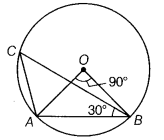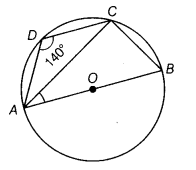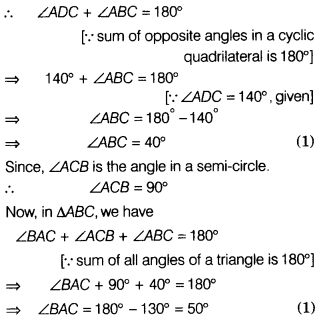# In the given figure, if ∠AOB = 90° and ∠ABC = 30°, then find the measure of ∠CAO

(i) In the given figure, if ∠AOB = 90° and
∠ABC = 30°, then find the measure of ∠CAO.(ii) If ABCD is a cyclic quadrilateral such that AB is a diameter of the circle circumscribing.it and ∠ADC = 140°, then find ∠BAC.

(i) We know that angle subtended at the centre is twice the angle subtended at any point on the remaining part of the circle.

(ii) Given, ABCD is a cyclic quadrilateral.